# signal_processing - Signal processing¶

Basic processing procedures for analog signals (e.g., performing a z-score of a signal, or filtering a signal).

elephant.signal_processing.butter(signal, highpass_freq=None, lowpass_freq=None, order=4, filter_function='filtfilt', fs=1.0, axis=-1)[source]

Butterworth filtering function for neo.AnalogSignal. Filter type is determined according to how values of highpass_freq and lowpass_freq are given (see Parameters section for details).

Parameters: signal : AnalogSignal or Quantity array or NumPy ndarray Time series data to be filtered. When given as Quantity array or NumPy ndarray, the sampling frequency should be given through the keyword argument fs. highpass_freq, lowpass_freq : Quantity or float High-pass and low-pass cut-off frequencies, respectively. When given as float, the given value is taken as frequency in Hz. Filter type is determined depending on values of these arguments: highpass_freq only (lowpass_freq = None): highpass filter lowpass_freq only (highpass_freq = None): lowpass filter highpass_freq < lowpass_freq: bandpass filter highpass_freq > lowpass_freq: bandstop filter order : int Order of Butterworth filter. Default is 4. filter_function : string Filtering function to be used. Either ‘filtfilt’ (scipy.signal.filtfilt()) or ‘lfilter’ (scipy.signal.lfilter()). In most applications ‘filtfilt’ should be used, because it doesn’t bring about phase shift due to filtering. Default is ‘filtfilt’. fs : Quantity or float The sampling frequency of the input time series. When given as float, its value is taken as frequency in Hz. When the input is given as neo AnalogSignal, its attribute is used to specify the sampling frequency and this parameter is ignored. Default is 1.0. axis : int Axis along which filter is applied. Default is -1. filtered_signal : AnalogSignal or Quantity array or NumPy ndarray Filtered input data. The shape and type is identical to those of the input.
elephant.signal_processing.cross_correlation_function(signal, ch_pairs, env=False, nlags=None)[source]

Computes unbiased estimator of the cross-correlation function.

Calculates the unbiased estimator of the cross-correlation function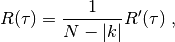where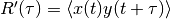in a pairwise manner, i.e. signal[ch_pairs[0,0]] vs signal2[ch_pairs[0,1]], signal[ch_pairs[1,0]] vs signal2[ch_pairs[1,1]], and so on. The cross-correlation function is obtained by scipy.signal.fftconvolve. Time series in signal are zscored beforehand. Alternatively returns the Hilbert envelope of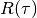, which is useful to determine the correlation length of oscillatory signals.

Parameters: signal : neo.AnalogSignal (nt x nch) Signal with nt number of samples that contains nch LFP channels ch_pairs : list (or array with shape (n,2)) list with n channel pairs for which to compute cross-correlation, each element of list must contain 2 channel indices env : bool Return Hilbert envelope of cross-correlation function Default: False nlags : int Defines number of lags for cross-correlation function. Float will be rounded to nearest integer. Number of samples of output is 2*nlags+1. If None, number of samples of output is equal to number of samples of input signal, namely nt Default: None cross_corr : neo.AnalogSgnal (2*nlag+1 x n) Pairwise cross-correlation functions for channel pairs given by ch_pairs. If env=True, the output is the Hilbert envelope of the pairwise cross-correlation function. This is helpful to compute the correlation length for oscillating cross-correlation functions ValueError If the input signal is not a neo.AnalogSignal. ValueError If ch_pairs is not a list of channel pair indices with shape (n,2). KeyError If keyword env is not a boolean. KeyError If nlags is not an integer or float larger than 0.

References

  (1, 2) Hall & River (2009) “Spectral Analysis of Signals, Spectral Element Method in Structural Dynamics”, Eq. 2.2.3

Examples

>>> dt = 0.02
>>> N = 2018
>>> f = 0.5
>>> t = np.arange(N)*dt
>>> x = np.zeros((N,2))
>>> x[:,0] = 0.2 * np.sin(2.*np.pi*f*t)
>>> x[:,1] = 5.3 * np.cos(2.*np.pi*f*t)
>>> # Generate neo.AnalogSignals from x
>>> signal = neo.AnalogSignal(x, units='mV', t_start=0.*pq.ms,
>>>     sampling_rate=1/dt*pq.Hz, dtype=float)
>>> rho = elephant.signal_processing.cross_correlation_function(
>>>     signal, [0,1], nlags=150)
>>> env = elephant.signal_processing.cross_correlation_function(
>>>     signal, [0,1], nlags=150, env=True)
>>> plt.plot(rho.times, rho)
>>> plt.plot(env.times, env) # should be equal to one
>>> plt.show()

elephant.signal_processing.hilbert(signal, N='nextpow')[source]

Apply a Hilbert transform to an AnalogSignal object in order to obtain its (complex) analytic signal.

The time series of the instantaneous angle and amplitude can be obtained as the angle (np.angle) and absolute value (np.abs) of the complex analytic signal, respectively.

By default, the function will zero-pad the signal to a length corresponding to the next higher power of 2. This will provide higher computational efficiency at the expense of memory. In addition, this circumvents a situation where for some specific choices of the length of the input, scipy.signal.hilbert() will not terminate.

Parameters: signal : neo.AnalogSignal Signal(s) to transform N : string or int Defines whether the signal is zero-padded. ‘none’: no padding ‘nextpow’: zero-pad to the next length that is a power of 2 int: directly specify the length to zero-pad to (indicates the number of Fourier components, see parameter N of scipy.signal.hilbert()). Default: ‘nextpow’. neo.AnalogSignal Contains the complex analytic signal(s) corresponding to the input signals. The unit of the analytic signal is dimensionless.
elephant.signal_processing.wavelet_transform(signal, freq, nco=6.0, fs=1.0, zero_padding=True)[source]

Compute the wavelet transform of a given signal with Morlet mother wavelet. The parametrization of the wavelet is based on .

Parameters: signal : neo.AnalogSignal or array_like Time series data to be wavelet-transformed. When multi-dimensional array_like is given, the time axis must be the last dimension of the array_like. freq : float or list of floats Center frequency of the Morlet wavelet in Hz. Multiple center frequencies can be given as a list, in which case the function computes the wavelet transforms for all the given frequencies at once. nco : float (optional) Size of the mother wavelet (approximate number of oscillation cycles within a wavelet; related to the wavelet number w as w ~ 2 pi nco / 6), as defined in . A larger nco value leads to a higher frequency resolution and a lower temporal resolution, and vice versa. Typically used values are in a range of 3 - 8, but one should be cautious when using a value smaller than ~ 6, in which case the admissibility of the wavelet is not ensured (cf. ). Default value is 6.0. fs : float (optional) Sampling rate of the input data in Hz. When signal is given as an AnalogSignal, the sampling frequency is taken from its attribute and this parameter is ignored. Default value is 1.0. zero_padding : bool (optional) Specifies whether the data length is extended to the least power of 2 greater than the original length, by padding zeros to the tail, for speeding up the computation. In the case of True, the extended part is cut out from the final result before returned, so that the output has the same length as the input. Default is True. signal_wt: complex array Wavelet transform of the input data. When freq was given as a list, the way how the wavelet transforms for different frequencies are returned depends on the input type. When the input was an AnalogSignal of shape (Nt, Nch), where Nt and Nch are the numbers of time points and channels, respectively, the returned array has a shape (Nt, Nch, Nf), where Nf = len(freq), such that the last dimension indexes the frequencies. When the input was an array_like of shape (a, b, …, c, Nt), the returned array has a shape (a, b, …, c, Nf, Nt), such that the second last dimension indexes the frequencies. To summarize, signal_wt.ndim = signal.ndim + 1, with the additional dimension in the last axis (for AnalogSignal input) or the second last axis (for array_like input) indexing the frequencies. ValueError If freq (or one of the values in freq when it is a list) is greater than the half of fs, or nco is not positive.

References

1. Le van Quyen et al. J Neurosci Meth 111:83-98 (2001)
2. Farge, Annu Rev Fluid Mech 24:395-458 (1992)
elephant.signal_processing.zscore(signal, inplace=True)[source]

Apply a z-score operation to one or several AnalogSignal objects.

The z-score operation subtracts the mean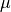of the signal, and divides by its standard deviation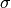: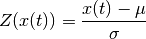If an AnalogSignal containing multiple signals is provided, the z-transform is always calculated for each signal individually.

If a list of AnalogSignal objects is supplied, the mean and standard deviation are calculated across all objects of the list. Thus, all list elements are z-transformed by the same values ofand. For AnalogSignals, each signal of the array is treated separately across list elements. Therefore, the number of signals must be identical for each AnalogSignal of the list.

Parameters: signal : neo.AnalogSignal or list of neo.AnalogSignal Signals for which to calculate the z-score. inplace : bool If True, the contents of the input signal(s) is replaced by the z-transformed signal. Otherwise, a copy of the original AnalogSignal(s) is returned. Default: True neo.AnalogSignal or list of neo.AnalogSignal The output format matches the input format: for each supplied AnalogSignal object a corresponding object is returned containing the z-transformed signal with the unit dimensionless.

Examples

>>> a = neo.AnalogSignal(
...       np.array([1, 2, 3, 4, 5, 6]).reshape(-1,1)*mV,
...       t_start=0*s, sampling_rate=1000*Hz)

>>> b = neo.AnalogSignal(
...       np.transpose([[1, 2, 3, 4, 5, 6], [11, 12, 13, 14, 15, 16]])*mV,
...       t_start=0*s, sampling_rate=1000*Hz)

>>> c = neo.AnalogSignal(
...       np.transpose([[21, 22, 23, 24, 25, 26], [31, 32, 33, 34, 35, 36]])*mV,
...       t_start=0*s, sampling_rate=1000*Hz)

>>> print zscore(a)
[[-1.46385011]
[-0.87831007]
[-0.29277002]
[ 0.29277002]
[ 0.87831007]
[ 1.46385011]] dimensionless

>>> print zscore(b)
[[-1.46385011 -1.46385011]
[-0.87831007 -0.87831007]
[-0.29277002 -0.29277002]
[ 0.29277002  0.29277002]
[ 0.87831007  0.87831007]
[ 1.46385011  1.46385011]] dimensionless

>>> print zscore([b,c])
[<AnalogSignal(array([[-1.11669108, -1.08361877],
[-1.0672076 , -1.04878252],
[-1.01772411, -1.01394628],
[-0.96824063, -0.97911003],
[-0.91875714, -0.94427378],
[-0.86927366, -0.90943753]]) * dimensionless, [0.0 s, 0.006 s],
sampling rate: 1000.0 Hz)>,
<AnalogSignal(array([[ 0.78170952,  0.84779261],
[ 0.86621866,  0.90728682],
[ 0.9507278 ,  0.96678104],
[ 1.03523694,  1.02627526],
[ 1.11974608,  1.08576948],
[ 1.20425521,  1.1452637 ]]) * dimensionless, [0.0 s, 0.006 s],
sampling rate: 1000.0 Hz)>]